International
Tables for
Crystallography
Volume D
Physical properties of crystals
Edited by A. Authier

International Tables for Crystallography (2006). Vol. D, ch. 1.1, pp. 16-17

## Section 1.1.4.7. Reduction of the components of a tensor of rank 2

A. Authiera*

aInstitut de Minéralogie et de la Physique des Milieux Condensés, Bâtiment 7, 140 rue de Lourmel, 75015 Paris, France
Correspondence e-mail: aauthier@wanadoo.fr

#### 1.1.4.7. Reduction of the components of a tensor of rank 2

| top | pdf |

The reduction is given for each of the 11 Laue classes.

#### 1.1.4.7.1. Triclinic system

| top | pdf |

Groups, 1: no reduction, the tensor has 9 independent components. The result is represented in the following symbolic way (Nye, 1957, 1985):where the sign • represents a nonzero component.

#### 1.1.4.7.2. Monoclinic system

| top | pdf |

Groups 2m, 2, m: it is sufficient to consider the twofold axis or the mirror. As the representative matrix is diagonal, the calculation is immediate. Taking the twofold axis to be parallel to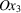, one has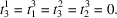The other components are not affected. The result is represented asThere are 5 independent components. If the twofold axis is taken along axis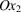, which is the usual case in crystallography, the table of independent components becomes#### 1.1.4.7.3. Orthorhombic system

| top | pdf |

Groups mmm, 2mm, 222: the reduction is obtained by considering two perpendicular twofold axes, parallel toand to, respectively. One obtainsThere are 3 independent components.

#### 1.1.4.7.4. Trigonal, tetragonal, hexagonal and cylindrical systems

| top | pdf |

We remarked in Section 1.1.4.6.2.3that, in the case of tensors of rank 2, the reduction is the same for threefold, fourfold or sixfold axes. It suffices therefore to perform the reduction for the tetragonal groups. That for the other systems follows automatically.

#### 1.1.4.7.4.1. Groups,;,,;,,;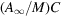,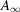| top | pdf |

If we consider a fourfold axis parallel torepresented by the matrix given in (1.1.4.3), by applying the direct inspection method one findswhere the symbol ⊖ means that the corresponding component is numerically equal to that to which it is linked, but of opposite sign. There are 3 independent components.

#### 1.1.4.7.4.2. Groups,,;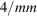,,,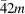;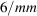,,,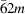;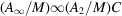,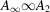| top | pdf |

The result is obtained by combining the preceding result and that corresponding to a twofold axis normal to the fourfold axis. One findsThere are 2 independent components.

#### 1.1.4.7.5. Cubic and spherical systems

| top | pdf |

The cubic system is characterized by the presence of threefold axes along the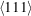directions. The action of a threefold axis along  on the componentsof a vector results in a permutation of these components, which become, respectively,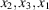and then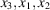. One deduces that the components of a tensor of rank 2 satisfy the relations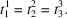The cubic groups all include as a subgroup the group 23 of which the generating elements are a twofold axis alongand a threefold axis along . If one combines the corresponding results, one deduces that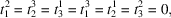which can be summarized byThere is a single independent component and the medium behaves like a property represented by a tensor of rank 2, like an isotropic medium.

#### 1.1.4.7.6. Symmetric tensors of rank 2

| top | pdf |

If the tensor is symmetric, the number of independent components is still reduced. One obtains the following, representing the nonzero components for the leading diagonal and for one half of the others.

#### 1.1.4.7.6.1. Triclinic system

| top | pdf |There are 6 independent components. It is possible to interpret the number of independent components of a tensor of rank 2 by considering the associated quadric, for instance the optical indicatrix. In the triclinic system, the quadric is any quadric. It is characterized by six parameters: the lengths of the three axes and the orientation of these axes relative to the crystallographic axes.

#### 1.1.4.7.6.2. Monoclinic system (twofold axis parallel to)

| top | pdf |There are 4 independent components. The quadric is still any quadric, but one of its axes coincides with the twofold axis of the monoclinic lattice. Four parameters are required: the lengths of the axes and one angle.

#### 1.1.4.7.6.3. Orthorhombic system

| top | pdf |There are 3 independent components. The quadric is any quadric, the axes of which coincide with the crystallographic axes. Only three parameters are required.

#### 1.1.4.7.6.4. Trigonal, tetragonal and hexagonal systems, isotropic groups

| top | pdf |There are 2 independent components. The quadric is of revolution. It is characterized by two parameters: the lengths of its two axes.

#### 1.1.4.7.6.5. Cubic system

| top | pdf |There is 1 independent component. The associated quadric is a sphere.

### References

Nye, J. F. (1957). Physical properties of crystals, 1st ed. Oxford: Clarendon Press.
Nye, J. F. (1985). Physical properties of cystals, revised ed. Oxford University Press.